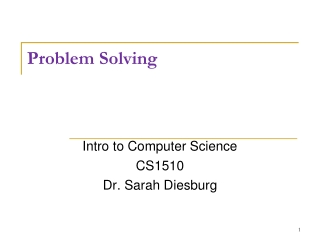DownloadDownload PresentationProblem Solving

# Problem Solving

Download Presentation## Problem Solving

- - - - - - - - - - - - - - - - - - - - - - - - - - - E N D - - - - - - - - - - - - - - - - - - - - - - - - - - -
##### Presentation Transcript

1. Problem Solving Intro to Computer Science CS1510 Dr. Sarah Diesburg

2. Numeric representation • We usually work with decimal numbers with digits from 0 to 9 and powers of 10 7313 = (7 * 1000 + 3 * 100 + 1 * 10 + 3 * 1) Or (7 * 103 + 3 * 102 + 1 * 101 + 3 * 100) • The binary number system uses digits 0 and 1 and powers of 2 0101 = (0 * 8 + 1 * 4 + 0 * 2 + 1 * 1) Or (0 * 23 + 1 * 22 + 0 * 21 + 1 *20) = 5

3. Thinking about this… • A nibble is half a byte • 0110 is one example • How many numbers can a nibble store? • Which numbers can a nibble store? • Largest nibble/smallest nibble?

4. Your turn #1 • What unsigned decimal numbers are represented by the following binary numbers? Example: 00000101 = 5 01000100 00001101 10110011

5. Your turn #2 • How would you write the following numbers in binary? Example: 14 = 8 + 4 + 2 -> 00001110 3 121 143

6. Encoding • Binary numbers can represent more things than just integers • Another example is ASCII • American Standard Code for Information Interchange • Character encoding scheme based on the English alphabet • http://en.wikipedia.org/wiki/ASCII

7. Programming in Python • We will be using Python3 • Install on your own computers or use one of the ITTC or Wright Hall labs • Can install from http://www.python.org • Walkthrough • Be sure to use version 3 (not version 2)!

8. Problem Solving • How do humans solve problems? • Once we know that, how to we translate that to how a computer would solve a problem? • Get in groups of two or three

9. Problem #1 • Suppose that on a recent trip, you left Cedar Falls with a full tank of gas and your odometer read 64783 miles. • When you returned, your odometer read 64969 miles. You refilled your gas tank with 8 gallons of gas. • What was your mileage per gallons (or MPG)?

10. Problem #1 • What is the answer? • How did you arrive at this specific answer? • What is the general purpose algorithm to solve this class of problem?

11. On Thursday and Friday • We are going to look at • How the computer does mathematics • How do we assign names to things • How do we put numbers in the computer and take them back out

12. For Lab Tomorrow • Start reading chapter 1